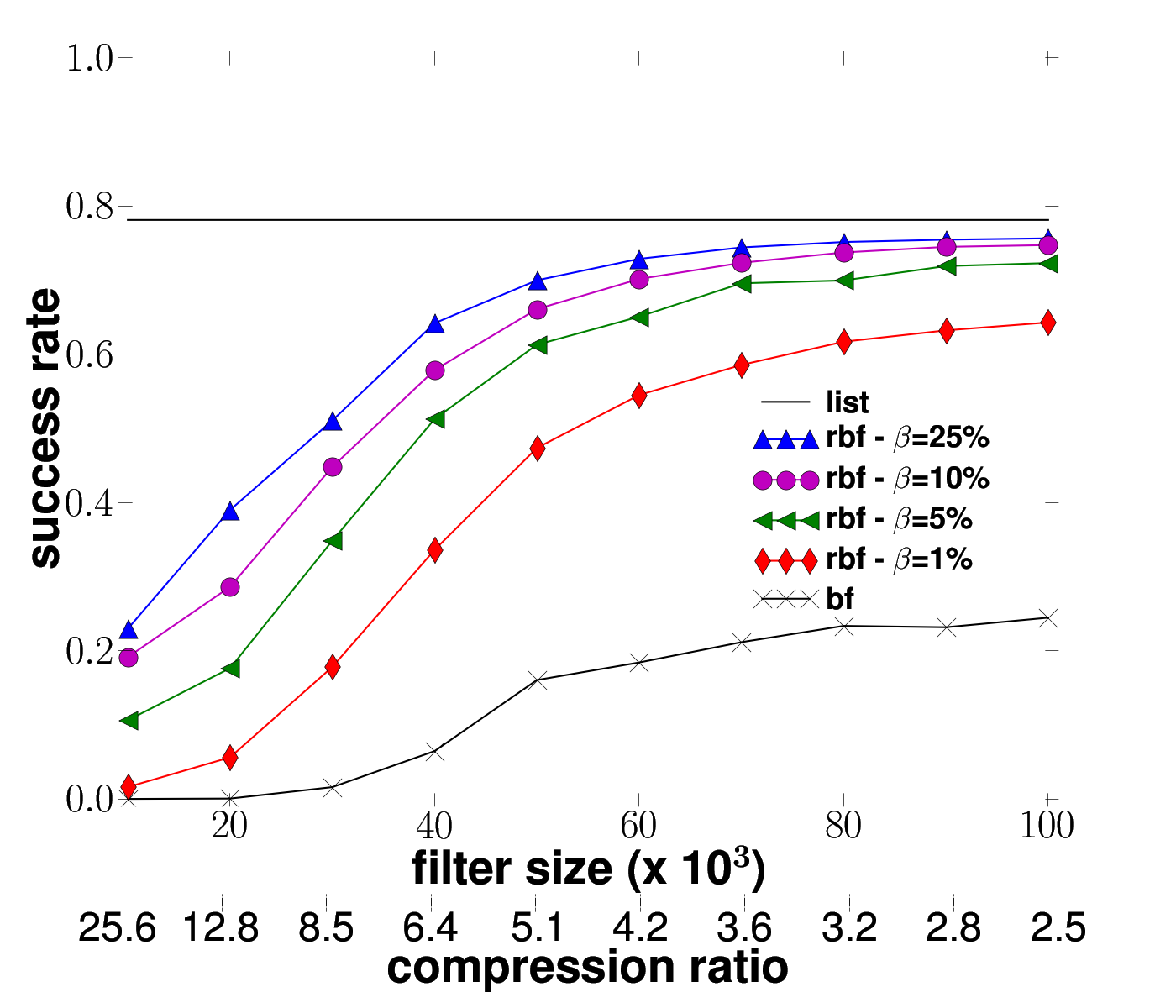# Complex Plot

Do you think it is possible to do such a plot in

> matplotlib?

Hey Benoit -- thanks for posting that image. It's good to get some
reminders of the current limitations of mpl so we can focus on
improving them. There are two things in that plot that mpl cannot
currently do.

1) multiple y-scales on the left and right. mpl supports a left and
right scale (see examples/two_scale.py) but nothing like an
arbitrary number of scales with arbitrary positioning as you see
in the plot you attached. This has long been on the wish list
(and is on the goals web page) but has not yet been implemented.
This is something chaco does quite well because it is very common
to make these plots in geophysics. For me it is one of the few
remaining must-have features for matplotlib 1.0. You could hack
your own scales using matplotlib lines and text instances. Indeed
it wouldn't be too hard and if I get some time I'll do a demo
which may serve as a prototype for refactoring the mpl axis code.

2) the horizontal legend layout - matplotlib is not very flexible in
the legend layout and supports only vertical legends. This would
be a nice feature. Currently the legend code can best be
described as hairy, which is why few want to try to improve it.

As for the text table at the bottom, you could either do this with
table, probably your best choice, or with a series of side-by-side
axes, or with some custom rectangles and text instances. We've talked
before that it would be nice to have a layout engine, much like the
gtk hbox and vbox, and this would be a good candidate for it.

JDH

Yo,

1) multiple y-scales on the left and right. mpl supports a left and
right scale (see examples/two_scale.py) but nothing like an
arbitrary number of scales with arbitrary positioning as you see
in the plot you attached. This has long been on the wish list
(and is on the goals web page) but has not yet been implemented.
This is something chaco does quite well because it is very common
to make these plots in geophysics. For me it is one of the few
remaining must-have features for matplotlib 1.0. You could hack
your own scales using matplotlib lines and text instances. Indeed
it wouldn't be too hard and if I get some time I'll do a demo
which may serve as a prototype for refactoring the mpl axis code.

I remember that, for a paper, I succeeded to plot two horizontal axis, on the bottom of the plot (see the image in attachment)

The difference with my previous mail is that the second axis (labeled 'compression ratio') is not used for plotting. It is, actually, the same than the other axis (labeled 'filter size') but it gives another scale/kind of information.

Here is my (very ugly) code:

from pylab import *
from matplotlib.font_manager import *

list = [0.78125356, 0.78125356, 0.78125356, 0.78125356, 0.78125356, 0.78125356, 0.78125356, 0.78125356, 0.78125356, 0.78125356]
yprops = dict(rotation=0, horizontalalignment='right',verticalalignment='center',x=-0.01)
axprops = dict(yticks=[])

rc('text', usetex=True)
rc('lines', markersize=16)
rc('legend', numpoints=3)

#getting data from different source files
xAxis = tabBF[:,0]
succBF = tabBF[:,1]
succRBF1 = tabRBF1[:,1]
succRBF2 = tabRBF2[:,1]
succRBF3 = tabRBF3[:,1]
succRBF4 = tabRBF4[:,1]

fig = figure(1)

#plot the figure
llist = ax1.plot(xAxis, list, 'k-', linewidth=1.5)
bf = ax1.plot(xAxis, succBF, 'k-x', linewidth=1.5)
rbf1 = ax1.plot(xAxis, succRBF1, 'r-d', linewidth=1.5)
rbf2 = ax1.plot(xAxis, succRBF2, 'b-^', linewidth=1.5)
rbf3 = ax1.plot(xAxis, succRBF3, 'g-<', linewidth=1.5)
rbf4 = ax1.plot(xAxis, succRBF4, 'm-o', linewidth=1.5)
axis([9, 101, -0.01, 1.01], font)
ax1.set_xlabel(r'\textbf{filter size (x 10}$^\mathbf{3}$\textbf{)}', font)
ax1.set_ylabel(r'\textbf{success rate}', font)
#ugly way of drawing the legend legend
leg = legend((llist, rbf2,rbf4, rbf3, rbf1, bf),(r'\textbf{list}', r'\textbf{rbf -} $\beta$\textbf{=25\%}', r'\textbf{rbf -} $\beta$\textbf{=10\%}',r'\textbf{rbf - }$\beta$\textbf{=5\%}', r'\textbf{rbf - }$\beta$\textbf{=1\%}', r'\textbf{bf}'), loc=(0.65,0.23), prop=FontProperties(size='26', weight='bold'))#leg = legend((llist, bf), (r'\textbf{list}', r'\textbf{bf}'), loc=(0.65,0.23), prop=FontProperties(size='26', weight='bold'))
leg.draw_frame(False)

#draw the second x-axis
This is clearly a hack (and I'm not very proud of it).